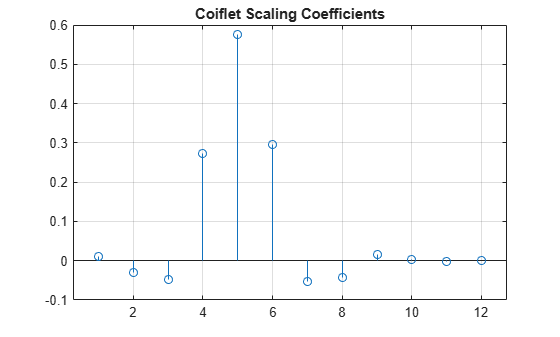# coifwavf

Coiflet wavelet filter

## Syntax

``f = coifwavf(wname)``

## Description

example

````f = coifwavf(wname)` returns the scaling filter `f` associated with the Coiflet wavelet specified by `wname`. `f` is a real-valued vector.```

## Examples

collapse all

Set the Coiflet wavelet name.

`wname = 'coif2';`

Compute and plot the scaling filter coefficients associated with the Coiflet.

```f = coifwavf(wname); stem(f) grid on title('Coiflet Scaling Coefficients')```## Input Arguments

collapse all

Name of Coiflet, specified as `'coifN'` where N is an integer between 1 and 5.

Example: `'coif3'`

## Version History

Introduced before R2006a